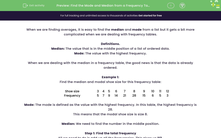# Find the Mode and Median from a Frequency Table

In this worksheet, students will practise finding the median and mode from frequency tables.Key stage:  KS 4

Year:  GCSE

GCSE Subjects:   Maths

GCSE Boards:   AQA, Eduqas, Pearson Edexcel, OCR,

Curriculum topic:   Statistics

Curriculum subtopic:   Statistics Analysing Data

Difficulty level:#### Worksheet Overview

When we are finding averages, it is easy to find the median and mode from a list but it gets a bit more complicated when we are dealing with frequency tables.

Definitions.

Median: The value that is in the middle position of a list of ordered data.

Mode: The value with the highest frequency.

When we are dealing with the median in a frequency table, the good news is that the data is already ordered.

Example 1:

Find the median and modal shoe size for this frequency table:

 Shoe size 3 4 5 6 7 8 9 10 11 12 Frequency 5 7 9 14 21 28 15 6 5 3

Mode: The mode is defined as the value with the highest frequency. In this table, the highest frequency is 28.

This means that the modal shoe size is size 8.

Median: We need to find the number in the middle position.

Step 1: Find the total frequency

All we need to do is add up all the frequencies. This gives us 113.

Step 2: Find the position of the median

To find the position of the median, we add 1 to the total and halve it:

(113 + 1) ÷ 2 = 57th

This means that the median is the 57th number in the list.

Step 3: Find the value of the median

To do this, the easiest way is to find the cumulative frequencies then see where the 57th number lies:

 Shoe size 3 4 5 6 7 8 9 10 11 12 Cumulative frequency 5 12 21 35 56 84 99 105 110 113

We can now see that shoe size 8 starts at the 57th number and ends at the 84th number.

The 57th number must, therefore, be in the shoe size 8 column.

Example 2: Find the median and modal groups for this grouped frequency table:

 Pocket money (p) 0 ≤ p < 10 10 ≤ p < 20 20 ≤ p < 30 30 ≤ p < 40 40 ≤ p < 50 50 ≤ p < 60 Frequency 4 6 11 10 8 6

The mode is defined as the value with the highest frequency. In this table, the highest frequency is 11.

This means the modal pocket money is 20 ≤ p < 30.

To find the median, we need to find the number in the middle position.

Step 1: Find the total frequency.

All we need to do is add up all the frequencies. This gives us 45.

Step 2: Find the position of the median

To find the position of the median, we add 1 to the total and halve it:

(45 + 1) ÷ 2 = 23

This means  that the median is the 23rd number in the list.

Step 3: Find the value of the median

To do this, the easiest way is to find the cumulative frequencies then see where the 23rd number lies:

 Pocket Money (p) 0 ≤ p < 10 10 ≤ p < 20 20 ≤ p < 30 30 ≤ p < 40 40 ≤ p < 50 50 ≤ p < 60 Frequency 4 6 11 10 8 6 Frequency (cum) 4 10 21 31 39 45

We can now see that 30 ≤ p < 40 starts at the 22nd number and ends at the 31st number.

The 23rd number must, therefore, be in the column 30 ≤ p < 40.

This is the median group.

Let's move on to some questions.

### What is EdPlace?

We're your National Curriculum aligned online education content provider helping each child succeed in English, maths and science from year 1 to GCSE. With an EdPlace account you’ll be able to track and measure progress, helping each child achieve their best. We build confidence and attainment by personalising each child’s learning at a level that suits them.

Get started